Courses

# Test: Heat (Competition Level 1)

## 30 Questions MCQ Test Physics Class 11 | Test: Heat (Competition Level 1)

Description
This mock test of Test: Heat (Competition Level 1) for Class 11 helps you for every Class 11 entrance exam. This contains 30 Multiple Choice Questions for Class 11 Test: Heat (Competition Level 1) (mcq) to study with solutions a complete question bank. The solved questions answers in this Test: Heat (Competition Level 1) quiz give you a good mix of easy questions and tough questions. Class 11 students definitely take this Test: Heat (Competition Level 1) exercise for a better result in the exam. You can find other Test: Heat (Competition Level 1) extra questions, long questions & short questions for Class 11 on EduRev as well by searching above.
QUESTION: 1

Solution:
QUESTION: 2

Solution:
QUESTION: 3

### How many minimum no. of C-atoms are required for position & geometrical isomerism in alkene?

Solution:
QUESTION: 4

How many structural formula are possible when one of the hydrogen is replaced by a chlorine atom in anthracene?

Solution:
QUESTION: 5

Which of the following connot be written in an isomeric form?

Solution:
QUESTION: 6

The number of cis-trans isomer possible for the following compound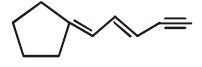Solution:
QUESTION: 7

The number of isomers of dibromoderivative of an alkene (molear mass 186 g mol–1) is

Solution:
QUESTION: 8

Increasing order of stability among the three main conformation (i.e. eclipse, anti, gauche) of ethylene glycol is:

Solution:
QUESTION: 9

How many primary amines are possible for the formula C4H11N?

Solution:
QUESTION: 10

The R/S configuration of these compounds are respectively.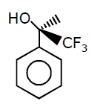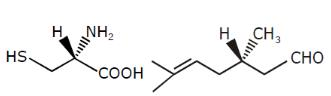Solution:
QUESTION: 11

How many planes (pos) are presents in Anthracene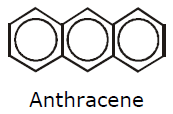Solution:
QUESTION: 12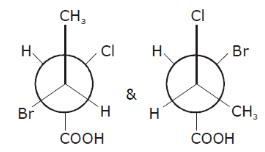Solution:
QUESTION: 13

Geometrical isomers can be

Solution:
QUESTION: 14

Phenol and benzyl alcohol are

Solution:
QUESTION: 15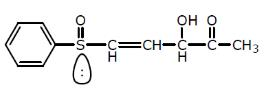Number of chiral centers are:

Solution:
QUESTION: 16

Which of the follownig compounds is (S)–4-chloro-1-methylcyclohexene ?

Solution:
QUESTION: 17

Which of the following compounds has two stereogenic centers (asymmetric carbons) ?

Solution:
QUESTION: 18

Which of the following structures represents a chiral compound ?

Solution:
QUESTION: 19

Examine the compound on the right. How many stereoisomers having this constitution are possible ?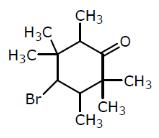Solution:
QUESTION: 20

Which of the following heptanols are chiral 1-heptanol, 2-heptanol, 3-heptanol, 4-heptanol.

Solution:
QUESTION: 21

Which of the following will exhibit geometrical isomerism ?

Solution:
QUESTION: 22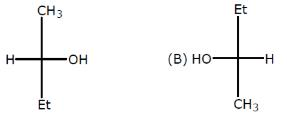Relation between given pair is

Solution:
QUESTION: 23

Following eclipsed form of propane is repeated after rotation of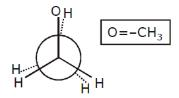Solution:
QUESTION: 24

Stereoisomers differ from each other in what respect ?

Solution:
QUESTION: 25

The number of isomers of C5H10 is

Solution:
QUESTION: 26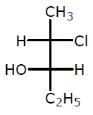The compound with the above configuration is called.

Solution:
QUESTION: 27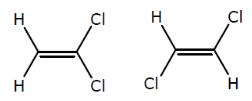The above compounds differ in

Solution:
QUESTION: 28

The compound C2H5OC2H5 and CH3OCH2CH2CH3 are

Solution:
QUESTION: 29

Which conformer of cyclohexane is chiral

Solution:
QUESTION: 30

Minimum C atoms required for a compound to show geometrical isomerism :

Solution: